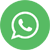• Learn the most popular programming languages: Python & R
• Avail JUMBO PASS
• Faculty are working Data Scientists, and Alumni of IIT, IIM, ISB & Ph.D.
• Live Projects - Internship opportunity with US-based firm for Students
• Special discounts for Students

# Machine Learning For Students

#### Prerequisite

Basic computer skills & Basic Mathematics

Python & R

40 hours

₹ 24000 ₹22000

## What Will I Learn?

Syllabus
##### Introduction to Python & R Programming
• Installation of Python & R Softwares
• Introduction to IDE's: Anaconda and RStudio
• Introduction to Important Features
• Decoding Packages and their usage
• Understand Pro’s and Con’s
• Introduction to Syntaxes
• Write your First Program using Python & R
• Hands-On
##### Basic Statistics
• Data types
• Probability
• Probability distribution
• Continuous Probability Distribution
• Discrete Probability Distribution
• Sampling Variation
• Inferential Statistics
• Sampling Techniques
• Probability Sampling
• Non-Probability Sampling
• Balanced / Unbalanced Data
• Graphical Representation of the Data
• Exploratory Data Analysis (EDA)
• Drawing Predictions - case study
• Interpretation of the Results
##### Data Mining
• What is Data Mining
• Stages of Analytics
• Descriptive Analytics
• Diagnostic Analytics
• Predictive Analytics
• Prescriptive Analytics
• Types of Machine Learning
• Supervised Learning
• Unsupervised Learning
• Reinforcement Learning
##### Data Mining Supervised Learning (Prediction Models)
• Bi-Variate Data Analysis
• Y = F(X) equation
• Prediction Models for a known Dependent Variable (Y)
• Regression Analysis
1. Linear Regression
• Scatter plot - Understanding the relationship between X and Y
• Correlation Coefficient (r) - Measure the relationship
• OLS - Technique to find the Best Fit Line for Prediction
• LINE assumptions
• Linearity
• Independency
• Normality
• Equal Variance
• Coefficient of determination (R^2)
• Hands-on: Predicting of Mileage of a Car
2. Logistic Regression
• Binomial distribution
• Confusion Matrix
• ROC curve and AUC
• Hands-on: Prediction of Attorney Hired or Not
##### Data Mining Supervised Learning (Classification Techniques)
• Prediction Models for a known Non-Numeric Dependent Variable (Y)
• Lazy learner - k-NN classifier
• Distance-based Classification Model
• Manual Calculations for Classifying Tomato into Vegetable/Fruit
• Identifying the best 'k' value
• Decision Tree (C5.0)
• Rules-based Classification technique
• Entropy
• Information Gained
• Manual Calculations of Information Gained to choose the Right Tree
• Introduction to Ensemble methods (Random Forest)
• Hands-on: Classifying a Bank Customer as a Good/Risky for Loan Approval/Rejection
• Black-Box Techniques: Artificial Neural Network
• Learn how Human Brain works
• Perceptron
• Multilayer Perceptron
• Integration Function
• Activation Function
• Weights Calculation in Neural Networks
• Hands-on: Prediction of Concrete Mixture Strength
##### Data Mining Unsupervised Learning
• Machine Learning Techniques where Dependent Variable is not Defined
• Clustering / Segmentation
• Grouping Homogenous Records
• Hierarchical/Agglomerative Clustering
• Distance Measures
• Dendrogram
• Cut-tree to get 'k' Clusters
• K-means
• Pre-defined 'k' Clusters
• The measure of Within Cluster similarity
• The measure of Between Cluster similarity
• Elbow Curve/Scree Plot - Find the Best value of 'k'
• Dimension Reduction
• PCA
• Reduction in the Number of Variables
• Visualization of High Dimensional Data in 2D
• Conditions to Measure the Weights
• PC's are in the Decreasing Order of Variance
• Correlation between any Pair of PC's is equal to Zero
• Sum of Squared Weights is equal to One
• Association Rules
• Probabilistic 'if-then' rules
• The measure of Strength for 'if-then' statements
• Support
• Confidence
• LiftRatio
• Apriori Algorithm
##### Text Mining
• Analyzing Text Data (Unstructured)
• Corpus / Document
• Pre-processing / Data Cleansing
• Stemming
• Tokenization
• Bag of Words
• DTM / TDM
• TFIDF
• Sentiment Analysis using Word Clouds
• Natural Language Processing
• Emotion Mining
• Web extraction - Amazon review's extraction## Why Take This Course?

The certification program of Data Science will cover the basics of statistics, Inferential statistics for predictive analytics, Graphical representation of Data to perform Descriptive Analytics, followed by Machine Learning models.

To make students/beginners to the world of analytics understand these business-oriented concepts we culminate the real-time case studies in the learning. Students will gain knowledge on understanding and defining Business problems, Steps in Data collection and Data Cleansing, Feature Engineering, Data Wrangling, Imputation, etc.

The ML for Beginners program is designed to suit students (graduation/post-graduation) or fresh pass outs who eventually want to venture into a career in analytics. Functionals oriented programming languages are used to discuss and implement the concepts of ML. These functional oriented programming languages are fun to learn and are super easy to grasp.

Hide

#### Enroll NowEnroll Now